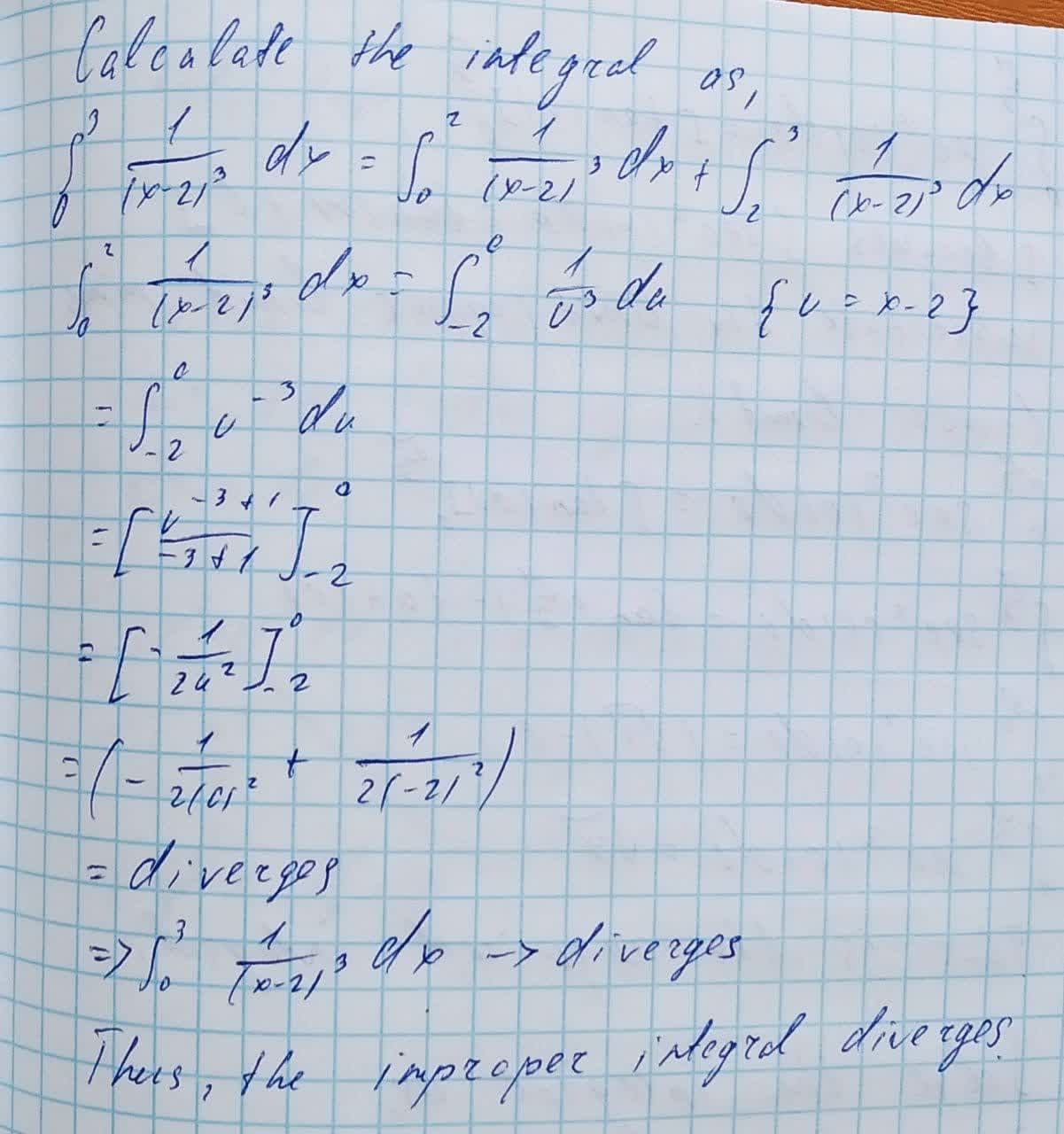Question# Does \int_{0}^{3}\frac{1}{(x-2)^{3}}dx improper integral converge?

Applications of integrals
ANSWEREDDoes the following improper integral converge?
$$\displaystyle{\int_{{{0}}}^{{{3}}}}{\frac{{{1}}}{{{\left({x}-{2}\right)}^{{{3}}}}}}{\left.{d}{x}\right.}$$